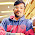Laws of Thermodynamics

Following are the three laws of thermodynamics : (Zeroth law of thermodynamics, First law of thermodynamics & Second law of thermodynamics).

1. Zeroth law of thermodynamics. This law states that when two bodies are in thermal equilibrium with a third body, they are also in thermal equilibrium with each other

2. First law of thermodynamics. This law states that the heat and mechanical work are mutually convertible. According to this law, a definite amount of mechanical work is needed to produce a definite amount of heat and vice versa.

This law also states that the energy can neither be created nor destroyed, though it can be transformed from one form to another. According to this law, the energy due to heat supplied (Q) must be balanced by the external workdone (W) plus the gain in internal energy (E) due to rise in temperature. In other words,

Q= W + E

3. Second law of thermodynamics.
This law states that there is a definite limit to the amount of mechanical energy, which can be obtained from a given quantity of heat energy.

According to Claussius, this law may be stated as "It is impossible for a self acting machine working in a cyclic process, to transfer heat from a body at a lower temperature to a body at a higher temperature without the aid of an external agency".

The second law of thermodynamics has also been stated by Kelvin-Planck as "It is impossible to construct an engine working on a cyclic process, whose sole purpose is to convert heat energy in to work." According to this statement, the second law of thermodynamics is sometimes called as law of degradation of energy.

1.can you provide the definitions with an example ?. So,that everyone can understand easily.

2.Third law is missing